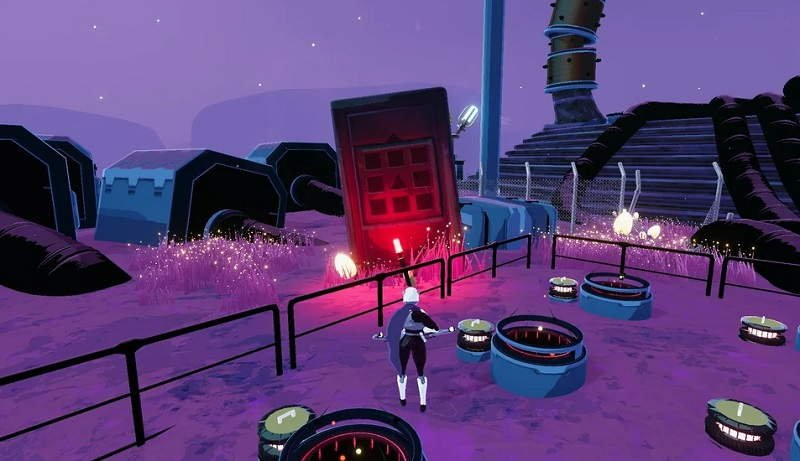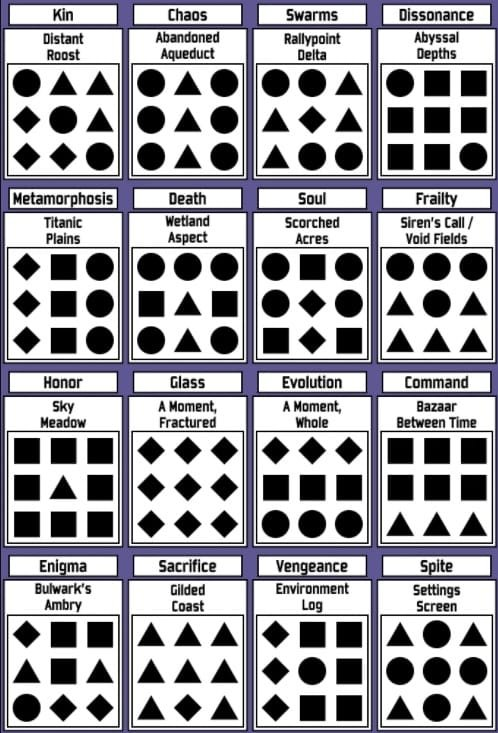Artifacts bring back the self-imposed cheats and challenges from Risk of Rain 2. While the technique for unlocking them differs from that of the original game, it isn’t as difficult if you know what you’re doing. Let’s go through all of the Risk of Rain 2 Artifacts codes and how to unlock them.

## How To Unlock Risk of Rain 2 Artifacts

Bulwark’s Ambry is a secret region where Artifacts can be found. To accomplish the challenge in Bulwark’s Ambry, you must collect an Artifact Key four times after entering the stage. When you pick one up, you must take it to the Artifact Reliquary in the middle, were interacting with it will lower its health bar by one-fourth. Enemies drop the Artifact Keys at random, so simply keep killing them until you get all four. The first key, which emerges when you enter the Hidden Realm, is the sole exception.## Risk of Rain 2 Artifacts Codes – All Artifacts

### Artifact of Chaos

• Effect: Friendly fire is enabled for survivors and monsters
• Code: Circle, Triangle, Circle – Circle, Triangle, Circle – Circle, Triangle, Circle

● ▲ ●
● ▲ ●
● ▲ ●

### Artifact of Command

• Code: Square, Square, Square – Square, Square, Square – Triangle, Triangle, Triangle

■ ■ ■
■ ■ ■
▲ ▲ ▲

### Artifact of Death

• Effect: When one player dies, everyone dies (useful for Ultimate Test)
• Code: Circle, Circle, Circle – Square, Triangle, Square – Circle, Triangle, Circle

●  ●  ●
■ ▲ ■
●  ▲  ●

### Artifact of Dissonance

• Effect: Monsters can appear outside their usual environments
• Code: Circle, Square, Square – Square, Square, Square – Square, Square, Circle

● ■ ■
■ ■ ■
■ ■ ●

### Artifact of Enigma

• Effect: Spawn a random equipment
• Code: Diamond, Square, Square – Triangle, Square, Triangle – Circle, Diamond, Diamond

♦ ■ ■
▲ ■ ▲
● ♦ ♦

### Artifact of Evolution

• Effect: Monsters gain items between stages
• Code: Diamond, Diamond, Diamond – Square, Square, Square – Circle, Circle, Circle

♦ ♦ ♦
■ ■ ■
● ● ●

### Artifact of Glass

• Effect: Allies deal 500% damage, but have 10% health
• Code: Diamond, Diamond, Diamond – Diamond, Diamond, Diamond – Diamond, Diamond, Diamond

♦ ♦ ♦
♦ ♦ ♦
♦ ♦ ♦

### Artifact of Frailty

• Effect: Fall damage is doubled and lethal
• Code: Circle, Circle, Circle –  Triangle, Circle, Triangle – Triangle, Triangle, Triangle

● ● ●
▲ ● ▲
▲ ▲ ▲

### Artifact of Honor

• Effect: Enemies can only spawn as elites
• Code: Square, Square, Square – Square, Triangle, Square – Square, Square, Square

■ ■ ■
■ ▲ ■
■ ■ ■

### Artifact of Kin

• Effect: Monsters will be of only one type per stage.
• Code: Circle, Triangle, Triangle – Diamond, Circle, Triangle – Diamond, Circle, Triangle

● ▲ ▲
♦ ● ▲
♦ ♦ ●

### Artifact of Metamorphosis

• Effect: Players always spawn as a random survivor
• Code: Diamond, Square, Circle – Diamond, Square, Circle – Diamond, Square, Circle

♦ ■ ●
♦ ■ ●
♦ ■ ●

### Artifact of Sacrifice

• Effect: Players always spawn as a random survivor
• Code: Diamond, Square, Circle – Diamond, Square, Circle – Diamond, Square, Circle

▲ ▲ ▲
▲ ▲ ▲
▲ ♦ ▲

### Artifact of Soul

• Effect: Monsters drop items on death, but Chests no longer spawn.
• Code: Triangle, Triangle, Triangle – Triangle, Triangle, Triangle – Triangle, Diamond, Triangle

● ■ ●
● ♦ ●
■ ♦ ■

### Artifact of Spite

• Effect: Enemies drop multiple exploding bombs on death
• Code: Triangle, Circle, Triangle – Circle, Circle, Circle – Triangle, Circle, Triangle

▲ ● ▲
● ● ●
▲ ● ▲

### Artifact of Swarms

• Effect: Monster spawns are doubled, but monster maximum health is halved
• Code: Circle, Circle, Triangle – Triangle, Diamond, Triangle – Triangle, Circle, Circle

● ● ▲
▲ ♦ ▲
▲ ● ●